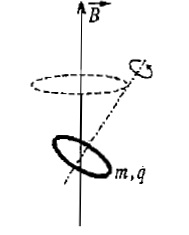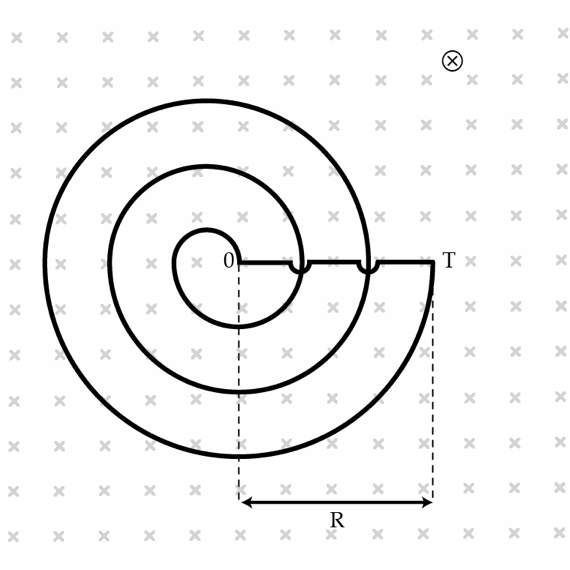Electricity and Magnetism

# Magnetic Flux, Induction, and Ampere's Circuital Law: Level 4-5 Challenges

Consider a uniformly charged circular disk of radius $R$ having charge $Q$ rotating about a fixed point on its circumference with an angular velocity $\omega$ . The magnetic field created at this point due to the motion is $k \times \dfrac{\mu_{0} Q \omega}{R}$. Find the value of $k$ .In homogenous magnetic field of induction $B$ thin charged ring is rotating around it's own axis. Mass of ring is $m$ and charge is $q$. Find the velocity of precession ring's axis around magnetic field line passing through the centar of ring.

$B=0.1T$

$q=3C$

$m=0.01kg$

One day, I was out bicycling when a thunderstorm cropped up. While frantically pedaling home at a speed of $10~\mbox{km/hr}$, a wind blew a power line down and it brushed against the inside of my front wheel, putting a positive $1~\mbox{C}$ charge on the wheel. I kept pedaling. If my bicycle wheels have radius $0.3~\mbox{m}$, what is the magnitude of the generated magnetic field in Teslas that I on my bike would measure at a distance of $0.1~\mbox{m}$ perpendicular from the center of my front wheel?

Details and assumptions

• Treat the wheels as thin conducting hoops.
• You may assume the charge has spread out over the wheel but not left it yet.
• My bike wheel was not slipping on the ground as I traveled.
• The vacuum permeability is $\mu_0=4\pi \times 10^{-7}~\mbox{H/m}$.

You probably know Gauss Law very well. It states that if you take any closed surface, the electric flux through this surface is proportional to the total charge enclosed. Mathematically: $\Phi_{E}:=\oint \vec{E} \cdot d\vec{A}=\frac{Q_{enc}}{\epsilon_{0}}.$ What about the magnetic flux? It turns out that $\Phi_{M}:=\oint \vec{B} \cdot d\vec{A}=0 \quad (\textrm{always!}).$ This is a Law of Nature, equivalent to one of Maxwell's equations and it reflects the experimental fact that there are no magnetic charges . In particular, $\Phi_{M}=0$ implies that not every magnetic field configuration can be realized in nature. For example, one can show that it is impossible to have a magnetic field that increases along the z-axis having only a z-component.

Consider an axially symmetric field with z-component (the field is symmetric about the z-axis) given by $B_{z}=B_{0}+ b z$ where $B_{0}= 2~ \mu \mbox{T}$ and $b=1 ~\mu \mbox{T/m}$.

Show that in addition to the z-component, this field must have a radial component $B_{r}$. Find $|B_{r}|$ in Teslas at a point located $50~\textrm{cm}$ away from the z-axis.

A wire is bent into the shape of an planar Archimedean spiral which in polar coordinates is described by the equation $r= b \theta.$ The spiral has $N=100$ turns and outer radius $R=10~\mbox{cm}$ ($R$ is the distance from point O to point T). Note that in the figure below we show a spiral having only 3 turns. The circuit is placed in a homogeneous magnetic field perpendicular to the plane of the spiral. The time dependence of the magnetic field induction is given by $B=B_{0}\cos(\omega t)$ where $B_{0}=1 ~\mu \mbox{T}$ and $\omega=2\times 10^{6}~\mbox{s}^{-1}$. Determine, the amplitude of the emf in Volts induced in the circuit.×